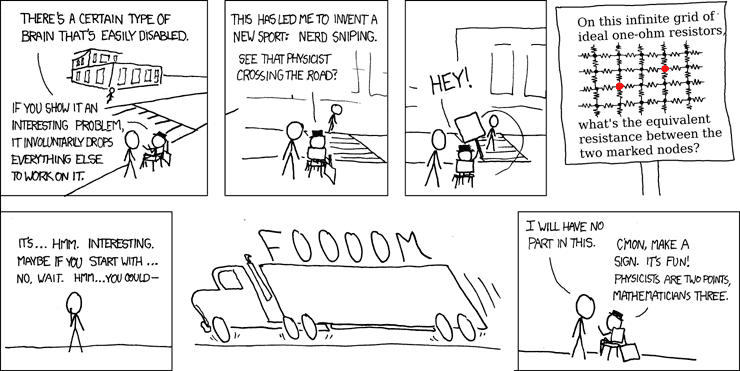# An infinite network (XKCD) - is it not clear?

OJFord
Hi, unless I'm missing something here, it seems to me that the answer is that it is infinite, and that that is pretty intuitive.

Is that not the case?I would think it could be simplified to view as two parallel and infinite resistances, giving ∞2/2∞, which simplifies to half infinity, which is of course really (in as much as it is) still infinite.

ZVdP
I have no solution, but the resistance cannot be larger than 1.5 Ohm, since there are two independent paths of 3 resistors between the points. The other parallel paths can only lower the resistance. So it's definitely finite.

The forum page of this comic will no doubt contain the correct answer.

Last edited:
Gold Member
I think it is intuitive that the resistance can not be infinite.

With some hand-waving: whenever you have a parallel circuit the equivalent resistance is lower than any of the branch resistance, so the resistance between any to adjacent nodes must be less than 1 Ohm. This means you have a path between the two marked nodes as a series of three networks that can be replaced with a resistor less than 1 Ohm, totaling less than 3 Ohm.

The exact solution, I seem to remember from years back, is a bit harder to obtain. Found a derivation  that may be of some use (haven't read it thoroughly enough to say if it is correct or not).

 http://mathpages.com/home/kmath668/kmath668.htm

OJFord
I have no solution, but the resistance cannot be larger than 1.5 Ohm, since there are two independent paths of 3 resistors between the points. The other parallel paths can only lower the resistance. So it's definitely finite.

I think it is intuitive that the resistance can not be infinite.

With some hand-waving: whenever you have a parallel circuit the equivalent resistance is lower than any of the branch resistance, so the resistance between any to adjacent nodes must be less than 1 Ohm.
 http://mathpages.com/home/kmath668/kmath668.htm

Right, that was stupid of me. Thank you both - and for the link.

I see why it was comic-worthy now, the answer is certainly not trivial!